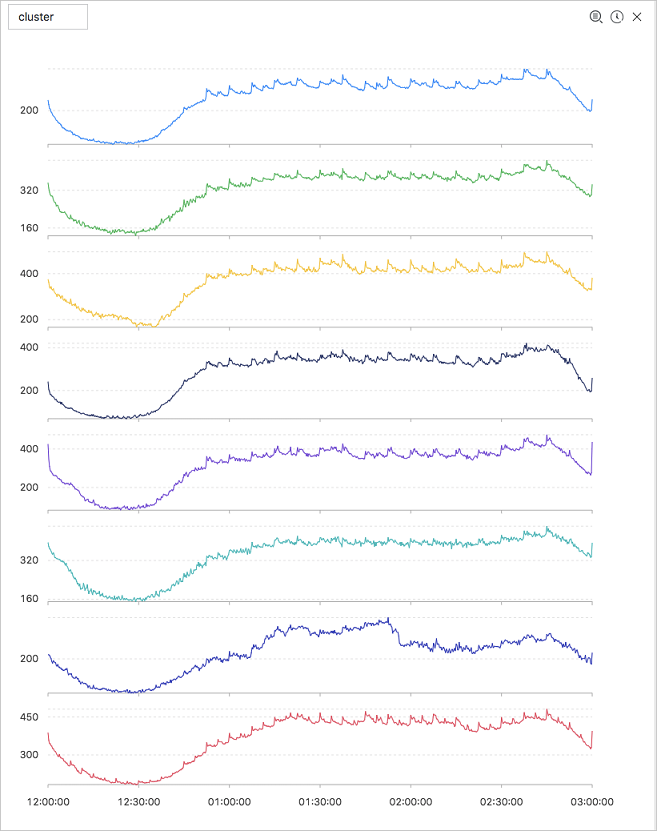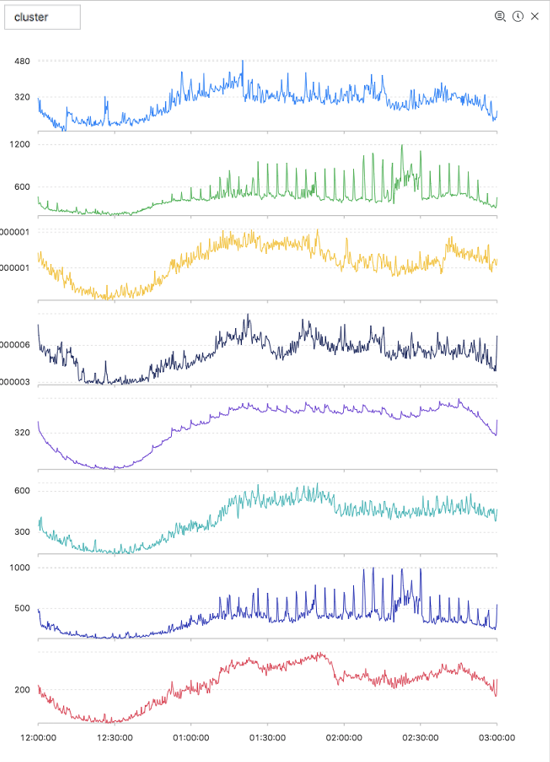This topic describes the time series clustering functions that you can use to cluster multiple pieces of time series data and obtain different curve shapes. Then, you can quickly find the corresponding cluster center and curves with shapes that are different from the curve shapes in the cluster.

## Function list

Function Description
`ts_density_cluster` Uses a density-based clustering method to cluster multiple pieces of time series data.
`ts_hierarchical_cluster` Uses a hierarchical clustering method to cluster multiple pieces of time series data.
`ts_similar_instance` Queries curves that are similar to a specified curve.

## ts_density_cluster

Function format:
``select ts_density_cluster(x, y, z) ``
The following table lists the parameters of the function.
Parameter Description Value
x The time sequence. The points in time along the horizontal axis are sorted in ascending order. Each point in time is a Unix timestamp. Unit: seconds.
y The sequence of numeric data corresponding to a specified point in time. N/A
z The name of the curve corresponding to the data at a specified point in time. The value is of the string type. Example: machine01.cpu_usr.
Example
• The query statement is as follows:
``* and (h: "machine_01" OR h: "machine_02" OR h : "machine_03") | select ts_density_cluster(stamp, metric_value,metric_name ) from ( select __time__ - __time__ % 600 as stamp, avg(v) as metric_value, h as metric_name from log GROUP BY stamp, metric_name order BY metric_name, stamp ) ``
• Output resultThe following table lists the display items.
Display item Description
cluster_id The category of the cluster. The value of -1 indicates that the cluster is not categorized in any cluster centers.
rate The proportion of instances in the cluster.
time_series The timestamp sequence of the cluster center.
data_series The data sequence of the cluster center.
instance_names The collection of instances included in the cluster center.
sim_instance The name of an instance in the cluster.

## ts_hierarchical_cluster

Function format:
``select ts_hierarchical_cluster(x, y, z) ``
The following table lists the parameters of the function.
Parameter Description Value
x The time sequence. The points in time along the horizontal axis are sorted in ascending order. Each point in time is a Unix timestamp. Unit: seconds.
y The sequence of numeric data corresponding to a specified point in time. N/A
z The name of the curve corresponding to the data at a specified point in time. The value is of the string type. Example: machine01.cpu_usr.
Example
• The query statement is as follows:
``* and (h: "machine_01" OR h: "machine_02" OR h : "machine_03") | select ts_hierarchical_cluster(stamp, metric_value, metric_name) from ( select __time__ - __time__ % 600 as stamp, avg(v) as metric_value, h as metric_name from log GROUP BY stamp, metric_name order BY metric_name, stamp )``
• Output resultThe following table lists the display items.
Display item Description
cluster_id The category of the cluster. The value of -1 indicates that the cluster is not categorized in any cluster centers.
rate The proportion of instances in the cluster.
time_series The timestamp sequence of the cluster center.
data_series The data sequence of the cluster center.
instance_names The collection of instances included in the cluster center.
sim_instance The name of an instance in the cluster.

## ts_similar_instance

Function format:
``select ts_similar_instance(x, y, z, instance_name, topK, metricType) ``
The following table lists the parameters of the function.
Parameter Description Value
x The time sequence. The points in time along the horizontal axis are sorted in ascending order. Each point in time is a Unix timestamp. Unit: seconds.
y The sequence of numeric data corresponding to a specified point in time. N/A
z The name of the curve corresponding to the data at a specified point in time. The value is of the string type. Example: machine01.cpu_usr.
instance_name The name of a specified curve to be queried in the z collection. The value is of the string type. Example: machine01.cpu_usr.
Note The curve to be queried must be an existing one.
topK The curves similar to the specified curve. A maximum of K curves are returned. N/A
metricType `{'shape', 'manhattan', 'euclidean'}`. The metric used to measure the similarity between time series curves. N/A
The query statement is as follows:
``* and m: NET and m: Tcp and (h: "nu4e01524.nu8" OR  h: "nu2i10267.nu8" OR  h : "nu4q10466.nu8") | select ts_similar_instance(stamp, metric_value, metric_name, 'nu4e01524.nu8' ) from ( select __time__ - __time__ % 600 as stamp, sum(v) as metric_value, h as metric_name from log GROUP BY stamp, metric_name order BY  metric_name, stamp )``
The following table lists the display items.
Display item Description
instance_name The list of metrics that are similar to the specified metric.
time_series The timestamp sequence of the cluster center.
data_series The data sequence of the cluster center.